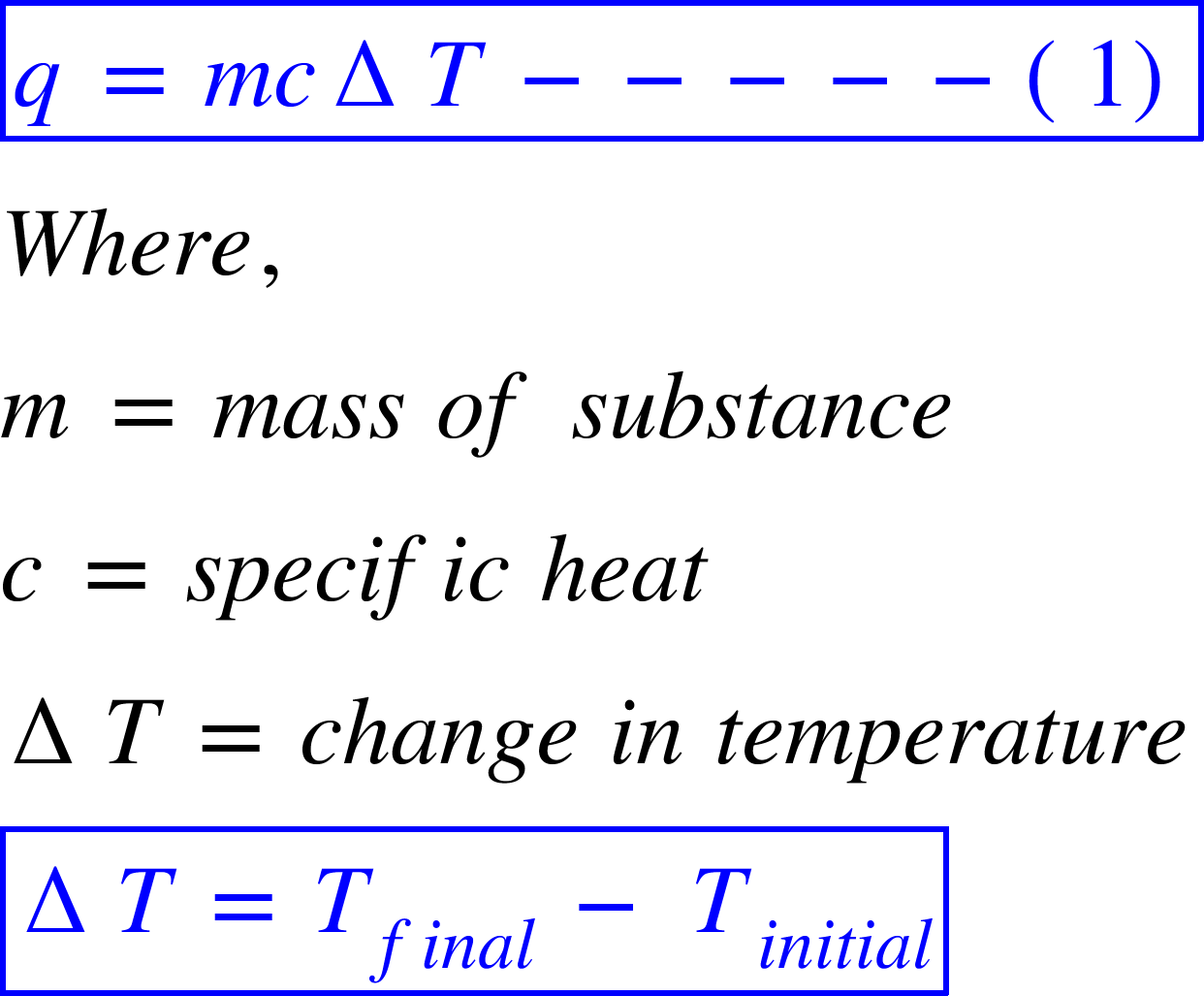# Problem: A swimming pool, 10.0 m by 4.0 m, is filled with water to a depth of 3.0 m at a temperature of 20.2°C. How much energy is required to raise the temperature of the water to 24.6°C?

###### FREE Expert Solution

This problem relates to specific heat capacity. It is the heat required to raise the temperature of 1 g of a substance by 1 oC. Note that the specific heat of water = 4.184 J/g/oC.

The amount of heat required to raise the temperature of a substance is given by the following formula:We want to raise the temperature of a swimming pool filled with water from 20.2 oC to 24.6 oC. So the value of ΔT is:

84% (295 ratings)###### Problem Details

A swimming pool, 10.0 m by 4.0 m, is filled with water to a depth of 3.0 m at a temperature of 20.2°C. How much energy is required to raise the temperature of the water to 24.6°C?